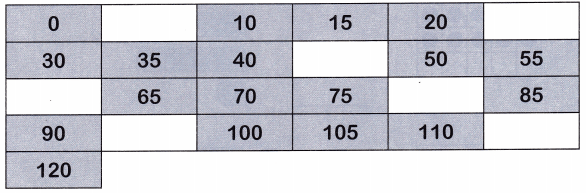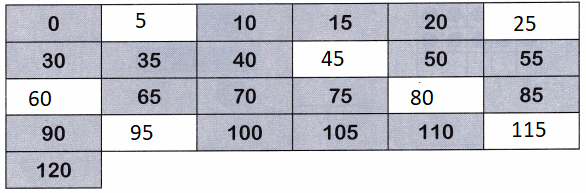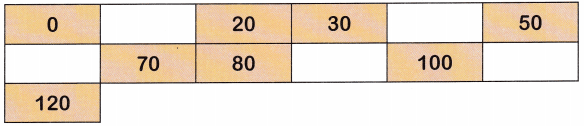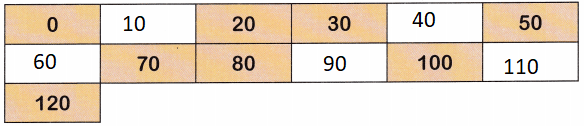All the solutions provided in McGraw Hill Math Grade 1 Answer Key PDF Chapter 6 Test as per the latest syllabus guidelines.

Count on. Write the missing numbers.

Count by 1s. Write the missing numbers.

Question 1.
103 104 ___ ___ ___
103 104 105 106 107
Explanation:
The given pattern is skip by ones
In skip by ones we have to add number 1
104 + 1 = 105
105 + 1 = 106

Question 2.
106 107 ___ ___ ___
106 107 108 109 110
Explanation:
The given pattern is skip by ones
In skip by ones we have to add number 1
107 + 1 = 108
108 + 1 = 109

Question 3.
114 115 ___ ___ ___
114 115 116 117 118
Explanation:
The given pattern is skip by ones
In skip by ones we have to add number 1
115 + 1 = 116
116 + 1 = 117

Question 4.
41 42 ___ ___ ___
41 42 43 44 45
Explanation:
The given pattern is skip by ones
In skip by ones we have to add number 1
42 + 1 = 43
43 + 1 = 44

Question 5.
56 57 ___ ___ ___
56 57 58 59 60
Explanation:
The given pattern is skip by ones
In skip by ones we have to add number 1
58 + 1 = 59
59 + 1 = 60

Question 6.
67 68 ___ ___ ___
67 68 69 70 71 72
Explanation:
The given pattern is skip by ones
In skip by ones we have to add number 1
69 + 1 = 70
70 + 1 = 71

Question 7.
97 98 ___ ___ ___
97 98 99 100 101
Explanation:
The given pattern is skip by ones
In skip by ones we have to add number 1
97 + 1 = 98
98 + 1 = 99

Question 8.
75 76 ___ ___ ___
75 76 77 78 79
Explanation:
The given pattern is skip by ones
In skip by ones we have to add number 1
76 + 1 = 77
77 + 1 = 78

Count by 10s. Write the missing numbers.

Question 9.
0 10 ___ ___ ___
0 10 20 30 40
Explanation:
The given series is skip by tens
we have to add number 10 the given number.
10 + 10 = 20
20 + 10 = 30

Question 10.
80 90 ___ ___ ___
80 90 100 110 120
Explanation:
The given series is skip by tens
we have to add number 10 the given number.
90 + 10 = 100
100 + 10 = 120

Question 11.
50 60 ___ ___ ___
50 60 70 80 90
Explanation:
The given series is skip by tens
we have to add number 10 the given number.
60 + 10 = 70
70 + 10 = 80

Question 12.
40 50 ___ ___ ___
40 50 60 70 80
Explanation:
The given series is skip by tens
we have to add number 10 the given number.
60 + 10 = 70
70 + 10 = 80

Question 13.
70 80 ___ ___ ___
70 80 90 100 110
Explanation:
The given series is skip by tens
we have to add number 10 the given number.
80 + 10 = 90
90 + 10 = 100

Question 14.
20 30 ___ ___ ___
20 30 40 50 60
Explanation:
The given series is skip by tens
we have to add number 10 the given number.
40 + 10 = 50
50 + 10 = 60

Question 15.
30 40 ___ ___ ___
30 40 50 60 70
Explanation:
The given series is skip by tens
we have to add number 10 the given number.
40 + 10 = 50
50 + 10 = 60

Question 16.
60 70 ___ ___ ___
60 70 80 90 100
Explanation:
The given series is skip by tens
we have to add number 10 the given number.
70 + 10 = 80
80 + 10 = 90

Count by 5s. Write the missing number.

Question 17.
25 30 ___ ___ ___
25 30 35 40 45
Explanation:
The given series is skip by tens
we have to add number 5 to the given number.
30 + 5 = 35
35 + 5 = 40

Question 18.
10 15 ___ ___ ___
10 15 20 25 30
Explanation:
The given series is skip by tens
we have to add number 5 to the given number.
15 + 5 = 20
20 + 5 = 25

Question 19.
65 70 ___ ___ ___
65 70 75 80 85
Explanation:
The given series is skip by tens
we have to add number 5 to the given number.
70 + 5 = 75
75 + 5 = 80

Question 20.
0 5 ___ ___ ___
0 5 10 15 20
Explanation:
The given series is skip by tens
we have to add number 5 to the given number.
5 + 5 = 10
10 + 5 = 15

Question 21.
80 85 ___ ___ ___
80 85 90 95 100
Explanation:
The given series is skip by tens
we have to add number 5 to the given number.
85 + 5 = 90
90 + 5 = 95

Question 22.
35 40 ___ ___ ___
35 40 45 50 55
Explanation:
The given series is skip by tens
we have to add number 5 to the given number.
40 + 5 = 45
45+ 5 = 50

Question 23.
100 105 ___ ___
100 105 110 115 120
Explanation:
The given series is skip by tens
we have to add number 5 to the given number.
105 + 5 = 110
110 + 5 = 115

Question 24.
15 20 ___ ___ ___
15 20 25 30 35
Explanation:
The given series is skip by tens
we have to add number 5 to the given number.
25 + 5 = 30
30 + 5 = 35

Question 25.
Count by 5s. Write the missing numbers.Explanation:
The given series is skip by tens
we have to add number 5 to the given number.

Question 26.
Count by 10s. Write the missing numbers.